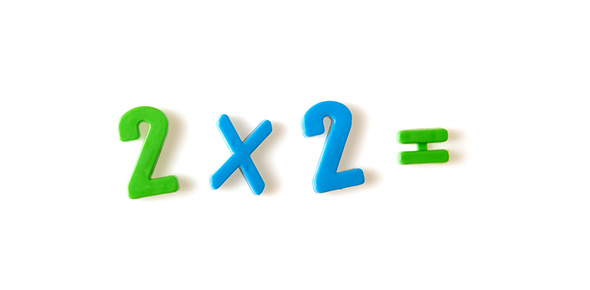# Multiplication Quiz: Basic Exam!

12 Questions | Total Attempts: 26Settings• 1.
2x2=
• 2.
2x11=
• 3.
2x3=
• 4.
2x1=
• 5.
2x5=
• 6.
2x6=
• 7.
2x9=
• 8.
2x4=
• 9.
2x10=
• 10.
2x7=
• 11.
2x8=
• 12.
2x12=
Related Topics